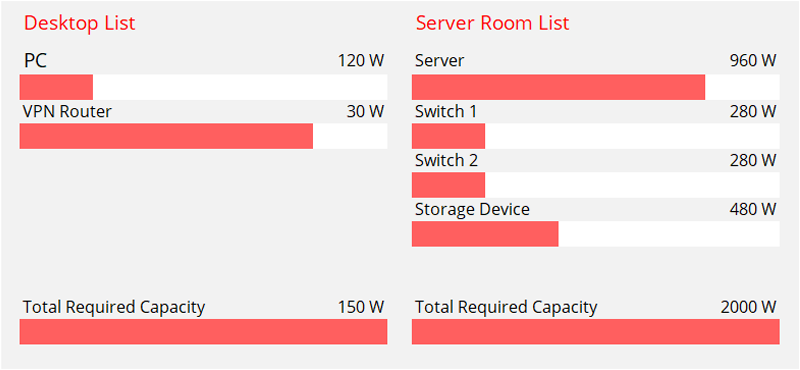Cancel

Change
English

### Explore

Change# How to Figure Out the Required UPS Capacity?

### Larry

June 2, 2020

Measured in “watts”, UPS capacity is an important factor to consider when choosing a UPS (uninterruptible power supply). It determines how many electronic devices the UPS system can support. This post will tell you how to choose the right UPS with required UPS capacity in the following four steps.

## Clarify UPS Measurement Units and Their Relationship

UPS systems are rated either in kilowatts (kW) or in kilo-volt-amperes (kVA). They can be regarded as the same in number. For example, in a direct current (DC) circuit, watts = volts x amps. In other words, 1 kW = 1 kVA.

However, they are not equal when the uninterruptible power supply system uses AC (alternating current). Normally, AC powers buildings and equipment in a more efficient way. Therefore, data centers usually use AC UPS power supplies. However, when hitting the transformer of the device, AC will exhibit reactive characteristics, which reduces the available power (watts) in apparent power (volt-amperes). The ratio of these two numbers is called the power factor (PF). Therefore, in AC circuits, watts = volts x amps x power factor. Power factors differ from each other in different scenarios. For example, large UPS systems are designed based on a power factor of 0.8, which means that a 100 kVA UPS can only support 80 kW of real power.

## Calculate the Maximum UPS Load

The load is the combined amount of power that electrical devices will consume. To calculate the load, one should make an equipment list, which includes the total watts each piece of equipment requires to run properly. For example, if you want to run a 120W PC, a 30W VPN router, a 960W server, two 280W network switches and a 480W storage device at the same time, the total load required is 2150 W.Note: If a piece of equipment has a redundant power supply, only count the wattage of one power supply.

## Estimate the Required UPS Capacity

Affected by power factors, the UPS is generally operated at about 80% of the actual rated capacity since the general PF is 0.8. That is to say, one only runs the uninterruptible power supply system around 80% of the capacity to support the load calculated. For example, if the total required capacity/load is 200 W, it is better to choose an UPS with a capacity of 250 W (250 W x 0.8 = 200 W) or so.

## Should One Choose an UPS Directly With Estimated UPS Capacity?

One may think that it is feasible to choose an UPS directly according to the estimated UPS capacity. Actually, it is not recommended to select the corresponding UPS based solely on the estimated UPS capacity. In addition to the estimated UPS capacity, two main factors, wiggle room and UPS runtime, should be taken into consideration.

### Wiggle Room

There would be no wiggle room at all if one bought a 1kVA UPS with a 900W UPS capacity (PF = 0.9) to support a calculated load of 900W. Under such circumstances, the whole system would be run at 100% capacity. Actually, regardless of how the PF ratings are stated (even if the PF is 1), a 100 kVA UPS will never support an actual full 100 kW load in the real world of the data center. It won’t be run at 100% capacity.

Since large UPS systems are three-phase, here let’s take a 100kVA UPS in a three-phase system with a 0.9 PF (90 kW capacity) as an example. Just as the table shows below, if Phase A is loaded to 95%, Phase B to 60% and Phase C to only 25%, the UPS will still have 40 kVA, or 36 kW, unused. Therefore, if the actual load required is 90 kW (100 kVA), a 90kW (100kVA) UPS is not recommended since it only offers an actual load of 54 kW (60 kVA). If one needs a full 900W load, it would be wise to get a 2kVA system to run it at 50% load capacity.### UPS Runtime

The actual UPS capacity required may also be affected by the UPS runtime in situations where more time for devices running is needed. For example, if the devices needed to be connected are on different floors or in offsite locations, the UPS must offer more time to keep the devices running. Otherwise, any failure caused by network downtime may result in immeasurable loss. Normally, there will be more runtime if the actual UPS capacity is much bigger than the required load. Imagine if a 1kVA/900W UPS offers 11 minutes of runtime at 100% load (900 W), one could use a 2kVA/1800W UPS from the same manufacturer running at 50% load (900 W) to get 24 minutes of runtime.

14.0k